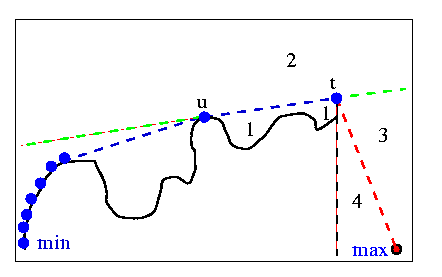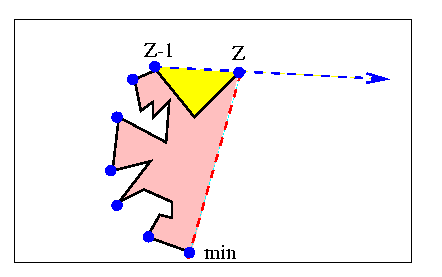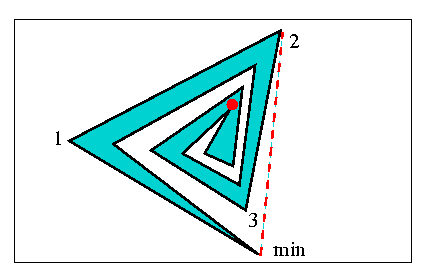Shin & Woo 1986

Shin and Woo proposed an algorithm  which employed "only one pocket test". Recall that the algorithms of Lee and Preparata and Shamos, among others, use a stack and divide the plane into regions when examining the next vertex to be potentially placed on the stack. All such algorithms that Shin and Woo make reference to use at least two pocket tests. The authors mention some of Orlowski's work, but they do not mention his algorithm of the previous section. This algorithm is identical to Orlowski's.

The figure below was used to describe the algorithm of Preparata and Shamos. Recall that there are two "pockets" (regions 1, 4) where their algorithm ignores vertices until one emerges into region 2 or 3. Essentially, the only way that Lee's algorithm is different is that in his algorithm the red dashed line extends back to the vertex labelled "min". These are the two pocket tests that Shin and Woo refer to.Shin and Woo ignore region 4. If you compare to Lee's algorithm, they ignore the pink region of the last figure. I edit that figure below to illustrate their algorithm. Suppose the polygon is oriented clockwise. Also suppose a stack contains a simple convex chain with potential hull points (blue), beginning at an extreme vertex (ex: Ymin). This chain is allowed to spiral. Let Z-1 and Z be the last two vertices on the stack. The only pocket defined (shown in yellow) is that which is to the right of the line (Z-1, Z), and to the left of the polygonal chain between Z-1 and Z. Shin and Woo do the following:

If the next vertex V after Z is in the yellow pocket, ignore it and all following vertices until one emerges from the pocket. Call this vertex V.

If V is to the right of the line (Z-1, Z) but not in the pocket, push V onto the stack.

If V is to the left of (Z-1, Z), delete the top of the stack until (Z-1, Z, V) form a convex turn. Then push V.

The difference from Lee's algorithm is that Shin and Woo don't check the pink pocket. As mentioned, this means that they allow the convex chain to spiral into the pink region. This is not a problem, since the polygon must start making concave turns to reach Ymin, so the backtracking step will take care of non-hull vertices.Obviously, the algorithm uses only one pocket test. I don't believe that this statement alone should imply that the algorithm is better. The extra cost of checking to see if the next vertex is in the pink region is insignificant. It may also save a lot of time, in the case of a spiraling polygon. The figure below illustrates this. Shin an Woo's algorithm will allow the convex chain to develop all the way into the middle at the red dot. Then it will go into the backtracking option over and over (although each iteration will be short). Lee's algorithm will simply ignore every vertex after 2.The nice thing about this work is that the pseudocode presented is actually quite a bit shorter than Lee's. They also provide a short PASCAL program to read in points and compute the hull.

previous : Orlowski 1985.          next : Werner 1986.